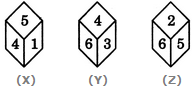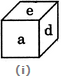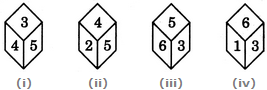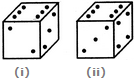# Non Verbal Reasoning - Cubes and Dice

### Exercise :: Cubes and Dice - Section 2

41.

Three different positions X, Y and Z of a dice are shown in the figures given below. Which of the hidden numbers adjacent to 5 in position X is/are common to the hidden numbers adjacent to 5 in position Z?A. 1 and 4 B. 2 C. 6 D. None

Answer: Option D

Explanation:

From positions X and Y we conclude that 1, 5, 6 and 3 lie adjacent to 4. Therefore, 2 must lie opposite 4. From positions Y and Z we conclude that 4, 3, 2 and 5 lie adjacent to 6. Therefore, 1 must lie opposite 6. Thus, 2 lies opposite 4, 1 lies opposite 6 and consequently 5 lies opposite 3.

Since 3 lies opposite 5 (as analysed above), it follows that 1, 4, 6 and 2 lie adjacent to 5. Out of these four numbers, the hidden numbers adjacent to 5 in position X are 6 and 2 and the hidden numbers adjacent to 5 in position Z are 1 and 4. Clearly, there is no number common.

42.

In a dice a, b, c and d are written on the adjacent faces, in a clockwise order and e and f at the top and bottom. When c is at the top, what will be at the bottom?A. a B. b C. c D. d

Answer: Option A

Explanation:

Clearly, the six faces are labelled as

Face I -> a, Face IV -> b, Face III -> c, Face II -> d, Face V -> e, Face VI -> f

Therefore 'a' appears opposite 'c'.

Hence, when 'c' is at the top, then 'a' will be at the bottom.

43.

Four usual dice are thrown on the ground. The total of numbers on the top faces of these four dice is 13 as the top faces showed 4, 3, 1 and 5 respectively. What is the total of the faces touching the ground?

 A. 12 B. 13 C. 15 D. Cannot be determined

Answer: Option C

Explanation:

In a usual dice, the sum of the numbers on any two opposite faces is always 7. Thus, 1 is opposite 6, 2 is opposite 5 and 3 is opposite 4.

Consequently, when 4, 3, 1 and 5 are the numbers on the top faces, then 3, 4, 6 and 2 respectively are the numbers on the face touching the ground. The total of these numbers = 3 + 4 + 6 + 2 = 15.

44.

The four different positions of a dice are given below: Find the number on the face opposite the face showing 6?A. 1 B. 2 C. 4 D. 5

Answer: Option C

Explanation:

From figures (i), (ii) and (iii), we conclude that 3, 4, 2 and 6 lie adjacent to 5. Therefore, 1 must lie opposite 5.

From figures (i), (iii) and (iv), we conclude that 4, 5, 6 and 1 lie adjacent to 3. Therefore, 2 must lie opposite 3. Now, we have 1 opposite 5 and 2 opposite 3. Hence, 4 must lie opposite 6.

45.

Two positions of a dice with 1 to 6 dots on its sides are shown below. If the dice is resting on the side with three dots, what will be number of dots on the side at the top?A. 1 or 5 B. 2 C. 3 D. 5

Answer: Option A

Explanation:

From figures (i) and (ii) we conclude that 2, 6 and 4 dots appear adjacent to 3 dots. Hence, either 1 or 5 dots may appear opposite 3 dots. Thus, if the dice is resting on the side with three dots, then the number of dots on the side at the top is either 1 or 5.

#### Current Affairs 2021

Interview Questions and Answers